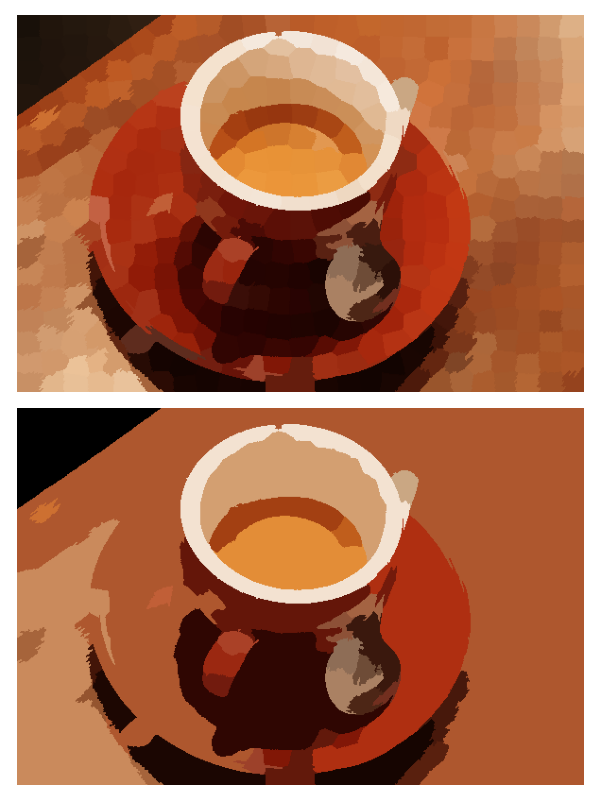# RAG Thresholding¶

This example constructs a Region Adjacency Graph (RAG) and merges regions which are similar in color. We construct a RAG and define edges as the difference in mean color. We then join regions with similar mean color.Out:

Clipping input data to the valid range for imshow with RGB data ([0..1] for floats or [0..255] for integers).
Clipping input data to the valid range for imshow with RGB data ([0..1] for floats or [0..255] for integers).


from skimage import data, segmentation, color
from skimage.future import graph
from matplotlib import pyplot as plt

img = data.coffee()

labels1 = segmentation.slic(img, compactness=30, n_segments=400, start_label=1)
out1 = color.label2rgb(labels1, img, kind='avg', bg_label=0)

g = graph.rag_mean_color(img, labels1)
labels2 = graph.cut_threshold(labels1, g, 29)
out2 = color.label2rgb(labels2, img, kind='avg', bg_label=0)

fig, ax = plt.subplots(nrows=2, sharex=True, sharey=True,
figsize=(6, 8))

ax.imshow(out1)
ax.imshow(out2)

for a in ax:
a.axis('off')

plt.tight_layout()


Total running time of the script: ( 0 minutes 1.969 seconds)

Gallery generated by Sphinx-Gallery